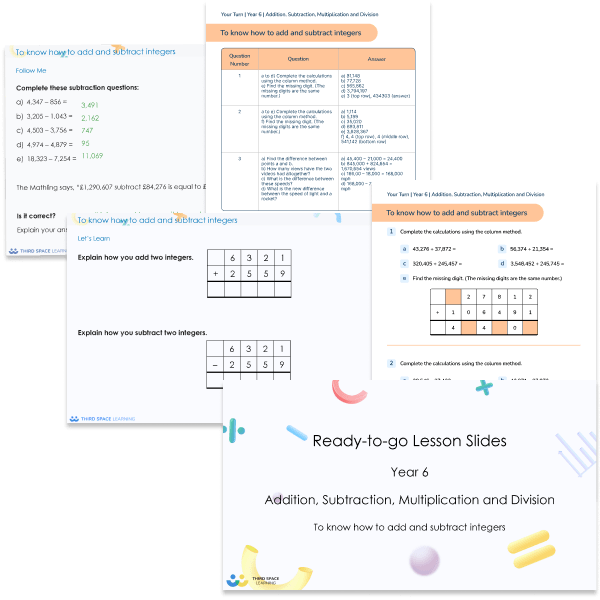##### Worksheets and Daily Practice, Whole Class Teaching

This addition, subtraction, multiplication and division Ready-to-go lessons pack for Year 6 includes 17 PowerPoints with matching worksheets ready for you to use with a class. These resources follow a mastery approach to mathematics teaching. The PowerPoints are fully editable with detailed notes for every teaching slide. Each associated worksheet is divided into three parts with a range of activities to complete across the learning area being covered.

This Year 6 addition, subtraction, multiplication and division pack contains 17 fully editable PowerPoints and 17 PDF worksheets.

These slides and worksheets cover:

• Common factors and multiples, prime numbers, square and cube numbers
• Rules of divisibility
• Multiplying and dividing using formal written methods
• Dividing using factors
• Problem solving involving the four operations
• Order of operations

Year 6

Multiplication, Addition, Division, Four operations, Subtraction